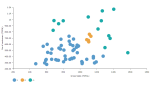# DCPY.DBSCANCLUST(min_samples, eps, columns)

The DBSCAN (Density Based Spatial Clustering of Applications with Noise) clustering algorithm views clusters as high-density areas separated by low density areas, when finding core samples and expanding clusters from them. Euclidean distance is used as a measure when calculating distance between data points.

###### Parameters
• min_samples – The number of data points in a neighborhood for a point to be considered as a core point, integer (default 5).

• eps – Maximum distance between two samples for them to be considered as the same neighborhood, float (default 0.5).

• columns – Dataset columns or custom calculations.

Example: DCPY.DBSCANCLUST(5, 0.5, sum([Gross Sales]), sum([No of customers])) used as a calculation for the Color field of the Scatterplot visualization.###### Input data
• Numeric variables are automatically scaled to zero mean and unit variance.
• Character variables are transformed to numeric values using one-hot encoding.
• Dates are treated as character variables, so they are also one-hot encoded.
• Size of input data is not limited, but many categories in character or date variables increase rapidly the dimensionality.
• Rows that contain missing values in any of their columns are dropped.
###### Result
• Column of integer values starting with 1, where each number corresponds to a cluster assigned to each record (row) by the algorithm. Data points that do not belong to any cluster are considered as noise (or outliers) and are assigned to -1.
• Rows that were dropped from input data due to containing missing value have missing value instead of assigned inlier/outlier value.
###### Key usage points
• Automatically estimates optimum number of clusters, which can be controlled with min_samples and eps parameters.
• Not all data points are assigned to a cluster. Data points that do not belong to any cluster are considered as noise (or outliers).
• Clusters can be of any shape, but should be of similar density.
• Can find clusters completely surrounded by different clusters.
• Robust towards outliers (noise).
• Sensitivity to order of the data.
• Does not work well if clusters vary in their density.
• Not scalable with number of records and memory usage inefficiency.
• Results are very sensitive to min_samples and eps parameters.
• Suffers from 'curse of dimensionality', which may result in misleading result when the number of variables is high.
###### Example

For the whole list of algorithms, see Data science built-in algorithms.